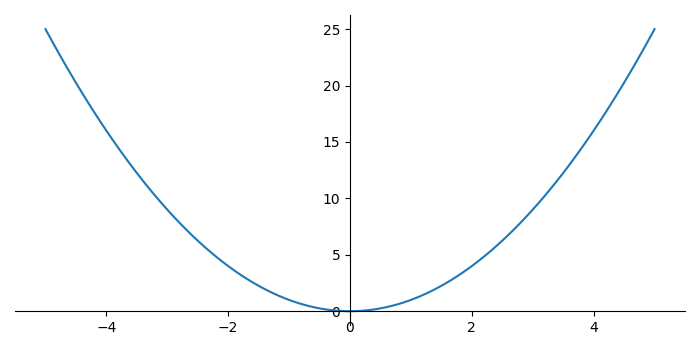# How to draw axis lines inside a plot in Matplotlib?

MatplotlibPythonData Visualization

To draw axis lines inside a plot in Matplotlib, we can take the following steps −

• Set the figure size and adjust the padding between and around the subplots.
• Create a new figure or activate an existing figure.
• Create x data points using numpy.
• Add an 'ax' to the figure as part of a subplot arrangement.
• Plot x and x**x data points using plot() method.
• Set the left and bottom positions at 0, whereas color of the right and top spines none.
• To display the figure, use show() method.

## Example

import numpy as np
import matplotlib.pyplot as plt

plt.rcParams["figure.figsize"] = [7.00, 3.50]
plt.rcParams["figure.autolayout"] = True

fig = plt.figure()

x = np.linspace(-5, 5, 100)

ax.plot(x, x*x)

ax.spines['left'].set_position('zero')
ax.spines['right'].set_color('none')
ax.spines['bottom'].set_position('zero')
ax.spines['top'].set_color('none')

plt.show()

## Output

It will produce the following output